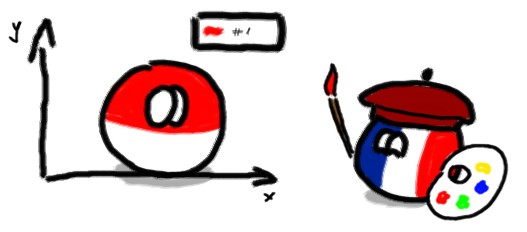Functions and figures in Matlab

This is a funny one – how to make a Matlab figure do some stuff on-demand with key presses and GUI elements.

Matlab R2017b – the MATLABWindow issue

I almost forgot, while discussing libpam last time, that the top reason for Matlab R2017b crashing in flames and destruction on Slackware 14.2 is actually MATLABWindow (and the Chromium underneath) that are causing problems. Solution? As always, cheat with libraries.

Matlab R2017b + Slackware 14.2 = libpam.so.0 missing

Installing newer versions of Matlab on not-PAM-ified linux requires supplying libpam.so.0 somewhere.

Beautiful plots in Latexhttps://github.com/matlab2tikz/matlab2tikz – Matlab to TikZ (Latex graphics) conversion tool for plots
https://www.sharelatex.com/learn/TikZ_package – TikZ documentation – general graphics
https://www.sharelatex.com/learn/Pgfplots_package – Pgfplots documentation – plotting tool inside Latex

Modifications and examples below.

Plot names in Matlab – using the ‘Tag’ property

Did you know you can tag the plots in Matlab for easier searching? Because I fuckin’ didn’t.

Why so brutal? Well, after 6 years of writing your PhD in Matlab you CAN become furious when you realise instead of keeping the links to the currently opened plots in a global variables and different lists you could’ve been naming them all that time…

Tag the plot: description for searching

aa = plot(2,2);
set(aa, 'Tag', 'foot trajectory' )
findobj('Tag','foot trajectory')
set( findobj('Tag','foot trajectory'), 'XData', [2 3], 'YData', '4 5')

… shit.

Matlab interface for Dynamixel motors and Bioloid robot

This is an old one, but is working quite nice and should be still useful for working with Dynamixel motor (AX-12+, AX-12A).
The goal was to have a nice toolbox used for direct control of Dynamixel motors using USB2Dynamixel or any other USB-Serial (half-duplex, ~1Mbps) adapter.